SYLLABUS  Previous: 5.2 Stochastic theory  Up: 5 MONTE-CARLO METHOD  Next: 5.4 A scheme for

## 5.3 Particle orbits

Slide : [ deterministic - Taylor - stochastic - Feynman-Kac || VIDEO login]

In the Monte Carlo method, the initial condition or indeed any function is discretized using quasi-particles; differential operators are therefore reformulated in terms of particle motions. Remember that a quasi-particle is defined in (1.4.7#eq.1) by a weight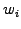, a position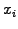and a shape function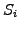; for simplicity, we assume here unit weights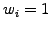and point shaped particles in the form of Dirac pulses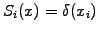. The solution can then be calculated from the particles density obtained from the positions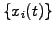called particle orbits. By construction, the lowest order moment is perfectly conserved, since no particle is lost.

To show the contrast between the Taylor and the Feynman-Kac theorems, which provide a rigorous foundation for the Monte Carlo method, the deterministic and the stochastic motion are first considered separately.Deterministic orbits
satisfy an ordinary differential equation for the position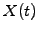as a function of time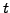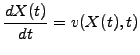(1)

or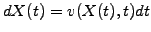(2)

This is solved numerically using the methods discussed in section 1.2.1, using an explicit or implicit discretization of time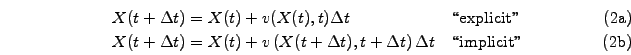For an ensemble of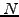particles with orbits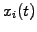, the particle density distribution function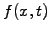is obtained by superposition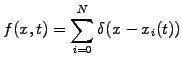(4)

and is plotted in the applet below using the projection (1.4.7#eq.3) from section 1.4.

 Numerical experiments: deterministic orbits Study in slow motion how the density function is obtained from a linear assignment on the coarse grid. Increase the number of Walkers=100, press Initialize and Start/Stop to verify that the lowest order moment is exactly conserved for all times. Increase the number of MeshPoints=64 and discuss whether the solution gets more precise. Increase to TimeStep=1 or any signed integer; verify that the deterministic motion of a cloud of particles provides in fact an exact description of advection once the initial condition has been discretized.

If a large number of particles approximate a smooth density function and each individual orbitevolves in a deterministic manner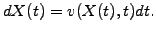(5)

then the Taylor's transport theorem states that the evolution of the particle density distribution functionsatisfies the advection equation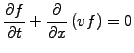(6)

The theorem makes it possible to study a PDE instead of an-particle system; the reverse is also true and will be used to solve the advection equation with particles.Stochastic particle orbits
are defined as an ensemble of possible orbits with different probabilities. Imagine a snowflake falling slowly from the sky: the motion is unpredictable and each evolution can be described with an orbitfollowing the stochastic differential equation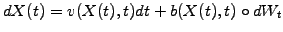(7)

where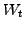is a Wiener processes (Brownian motion). Averaging over a large number of orbits, the average velocity and the broadening of possible orbits are readily obtained (exercise 5.02) as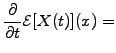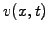(8)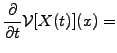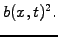(9)

Stochastic orbits can therefore be parametrized with statistical averages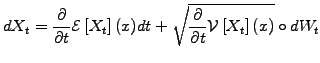(10)

and lead to a different result each time the JBONE applet is executed.

 Numerical experiments: stochastic orbits Take a few single Step 1 and convince yourself that the direction where the particle moves does not depend on the past. Re-Initialize and verify that the same stochastic differential equation each time produces a different orbit. Increase the number of Walkers=100 and observe how the cloud of independent particles diffuses in time. Vary the Velocity and Diffusion parameters, which are linked to the average velocityand the broadening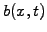of the orbits.

In the same spirit as in Taylor's transport theorem, it is possible to relate the evolution of a large number of stochastic particle orbits to a PDE; the so-called Feynman-Kac theorem tells that a smooth density distribution functionin which individual particles move according to(11)

evolves according to the Fokker-Planck equation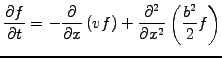(12)

which is in fact an advection-diffusion equation.

For example, letbe a stochastic process with an evolution of the probability density distributionfollowing the advection-diffusion equation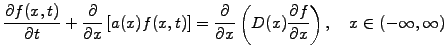(13)

From the Feynman-Kac theorem, it is easy to show (exercise 5.01) thatsatisfies the stochastic differential equationshowing exactly what evolution is required to reproduce statistically the properties desired at the macroscopic scale.

SYLLABUS  Previous: 5.2 Stochastic theory  Up: 5 MONTE-CARLO METHOD  Next: 5.4 A scheme for